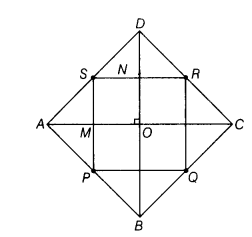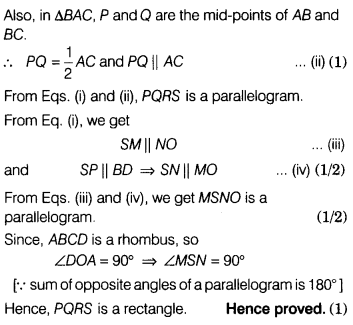# Prove that the quadrilateral PQRS is a rectangle

ABCD is a rhombus and P,Q, R and S are the mid-points of AB, BC, CD and DA respectively. Prove that the quadrilateral PQRS is a rectangle.

Given ABCD is a rhombus, and P, Q, R and S are the mid-points of AB, BC, CD and DA, respectively.To prove PQRS is a rectangle.
Construction Join AC and BD
Proof In ∆ DAC,S and R are the mid-points of AD and DC.